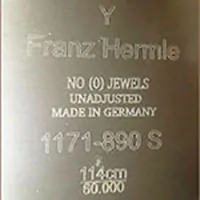# hermle-dates

July 14, 2019 8:31 am Leave your thoughts### Hermle date codes, what year it was madeEarly movements prior to the year 1987 have just the stamp of the year on the back of the clock movement, 81 just means 1981. From 1987 on, they used letter codes instead of numbers to represent the year made. Such as the year 1987 is only marked with the letter A instead, and 1988 is the letter B.
 A = 1987 B = 1988 C = 1989 D = 1990 E = 1991 F = 1992 G = 1993 H = 1994 G = 1995 H = 1996 I = 1997 J = 1998 K = 1999 L = 2000 M = 2001 N = 2002 O = 2003 P = 2004 Q = 2005 R = 2006 S = 2007 T = 2008 U = 2009 V = 2010 W = 2011 x = 2012 y = 2013 Z = 2014 AA = 2015 BB = 2016 CC = 2017 DD = 2018 EE = 2019